Courses

# Statistical Physics MSQ

## 10 Questions MCQ Test Topic wise Tests for IIT JAM Physics | Statistical Physics MSQ

Description
This mock test of Statistical Physics MSQ for IIT JAM helps you for every IIT JAM entrance exam. This contains 10 Multiple Choice Questions for IIT JAM Statistical Physics MSQ (mcq) to study with solutions a complete question bank. The solved questions answers in this Statistical Physics MSQ quiz give you a good mix of easy questions and tough questions. IIT JAM students definitely take this Statistical Physics MSQ exercise for a better result in the exam. You can find other Statistical Physics MSQ extra questions, long questions & short questions for IIT JAM on EduRev as well by searching above.
*Multiple options can be correct
QUESTION: 1

### Five identifiable particles are distributed in three non-degenerate levels with energies 0, E and 2E. The most probable distributions for total energy, 3E corresponds to what combination of options given below? Select one or more:

Solution:

As the levels are non-degenerate, there is only one state for each energy.
Let the number of particles occupying the 3 energy states be N1, N2, and Nrespectively
where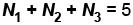The particles are identifiable, the number of ways of choosing the particles is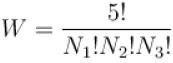The energy of system is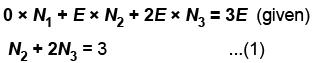Now, the most probable distribution is the one in which W is a maximum, subject to constraint given by equation (1)
Thus if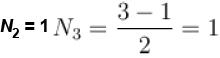and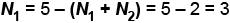If N2 = 3, N3 = 0
and N1 = 5 – (3 + 0) = 2
No other distribution are possible
For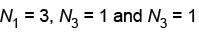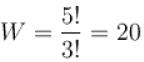So must probable distribution is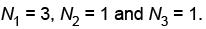The correct answers are: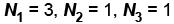*Multiple options can be correct
QUESTION: 2

### In relation to statistical mechanics choose the correct statements. Select one or more:

Solution:

For a given kind, all particles are exactly the same and are thus indistinguishable.
For each degree of freedom, there is one position coordinate and one canonical momentum,
∴ for n degrees, 2n will be the dimension.
The correct answers are: All particles of given kind are treated as mutually indistinguishable., The phase space for n degrees of freedom will have 2n dimensions and it unit cell volume in nn.

*Multiple options can be correct
QUESTION: 3

### Degeneracy in perfect gases is the departure of their properties from those of ordinary gas and becomes significant at : Select one or more:

Solution:

At very low temperature/high energy densities, the concentration of particles with similar momentum (low) would violate the Pauli’s exclusion principle.
The correct answers are: Very low temperature, High density

*Multiple options can be correct
QUESTION: 4

Choose the correct alternatives :
Select one or more:

Solution:

Bosons are particles that carry force, there is an attractive force between bosons (except for photons carrier of electromagnetic force, they can be repulsive also.)
Fermions are particles that make up the matter, they due to Pauli’s exclusion principle exert repulsive force on each other.
It is due to these respective attractions and repulsions that at given temperature.
Bose gas has lower pressure as compared to both Fermi gas and classical gas and Fermi gas has higher pressure.
The correct answers are: A fermion gas will exert greatest pressure, A boson gas will exert the least pressure.

*Multiple options can be correct
QUESTION: 5

In a grand canonical ensemble, a system A fixed volume is contact with a large reservoir B. Then
Select one or more:

Solution:

A grand canonical ensemble is such that the system can exchange energy and particles with a reservoir so that various possible states of the system can differ in both then total energy and total number of particles.
The correct answers are: A can exchange energy with B, A can exchange particles with B

*Multiple options can be correct
QUESTION: 6

Which of the following statements are true :
Select one or more:

Solution:

For system following F-D statistics, there is a limit on the number of particles occupying a certain energy level. This comes due to the fact that they follow Pauli’s exclusion principle, hence the particles, are distinguishable. Pauli’s exclusion principle on the other hand is not followed by system with B-E statistics and M-B statistics, hence the particles here are considered to be indistinguishable.
The correct answers are: particles following F-D statistics are distinguishable, Particles following B-E statistics are indistinguishable, Particles following Maxwell Boltzmann statistics are indistinguishable.

*Multiple options can be correct
QUESTION: 7

In a canonical ensemble
Select one or more:

Solution:

In a canonical ensemble, system can exchange only energy with environment.
Number of particles remains constant, also volume of the system remains constant. Therefore, temperature and density of system are constant.
The correct answers are: temperature is constant, density is constant

*Multiple options can be correct
QUESTION: 8

Liouville’s theorem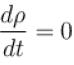holds for :
Select one or more:

Solution:

Liouville’s theorem is all about the conservation of density of points ρ is phase space.
i.e.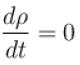⇒ ρ is conserved.
Also we know that in microcanonical ensemble, the system is isolated in the sense that it cannot exchange energy or particles with its environment.
Hence Liouville’s theorem holds only for microcanical ensemble.
The correct answer is: micro canonical ensemble

*Multiple options can be correct
QUESTION: 9

The ensemble average of a physical quantity is equal to its time average. This is true for :
Select one or more:

Solution:

The ensemble average of any physical quantity is equal to its time average and holds for all the ensembles whether micro-canonical, canonical or grand canonical ensemble.
The correct answers are: a microcanonical ensemble, canonical ensemble, grand canonical ensemble

*Multiple options can be correct
QUESTION: 10

Electronic contribution of specific heat varies linearly with the temperature for :
Select one or more:

Solution:

Electronic contribution of specific heat is completely independent of the dimension of the system and is always linear with the temperature.
The correct answers are: 3-dimensional electron gas, 2-dimensional electron gas, 1-dimensional electron gas